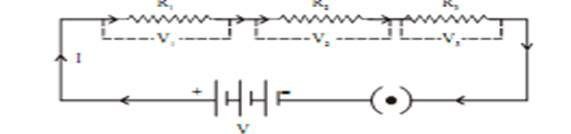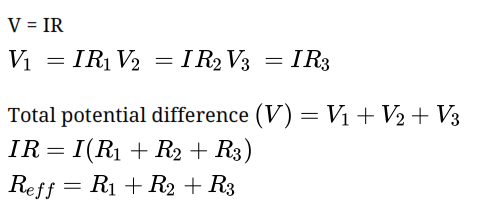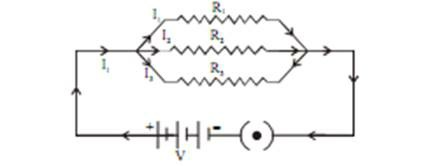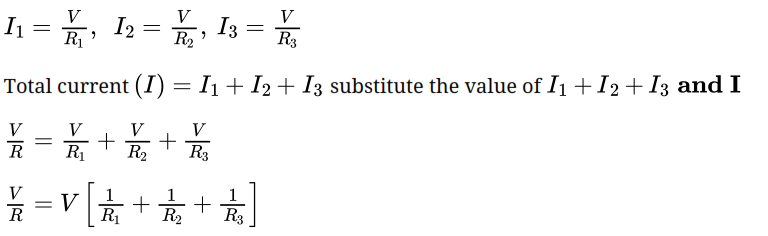# Resistance in Series (Maximum Effective Resistance)

Let us take three conductors/resistors of resistance R1, R2 and R3 that areconnected in series in a circuit.Ohm’s law stated that the current (I) flowing through the resistance in series will remain the same, whereas the potential difference (V) across each resistor will be different.Thus, we conclude that effective Resistance of the several resistors joined in series is equal to the algebraic sum of their individual resistances. Also, the net resistance increases when resistors are connected in series.

# Resistance in Parallel (Minimum Effective Resistance)

Let us take three resistors of resistance that are connected in parallel to the electric circuit.Thus, we conclude that the reciprocal of total effective resistance of the several resistors connected in parallel is equal to the sum of the reciprocals of the individual resistances. Also, the net resistance reduces when resistors are connected in parallel.

# The disadvantage of Series Connection in an Electric Circuit

• In a series connection, if any of the components fail to work, the circuit will break and then none of the component (ex. TV, bulb, fan..) will work.
• It is not possible to connect a bulb and a heater in series, because they need the different value of current to operate properly.

Hence, to overcome this problem we generally use the parallel circuit.